The radioactive half-life for a given radioisotope is a measure of the tendency of the nucleus to "decay" or "disintegrate" and as such is based purely upon that probability. The tiny nuclear size compared to the atom and the enormity of the forces which act within it make it almost totally impervious to the outside world. The half-life is independent of the physical state (solid, liquid, gas), temperature, pressure, the chemical compound in which the nucleus finds itself, and essentially any other outside influence. It is independent of the chemistry of the atomic surface, and independent of the ordinary physical factors of the outside world. The only thing which can alter the half-life is direct nuclear interaction with a particle from outside, e.g., a high energy collision in an accelerator.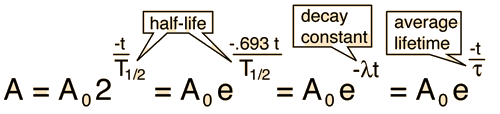Show

The predictions of decay can be stated in terms of the half-life , the decay constant, or the average lifetime. The relationship between these quantities is as follows.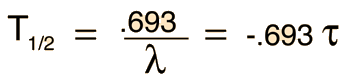Note that the radioactive half-life is not the same as the average lifetime, the half-life being 0.693 times the average lifetime. The example that causes the most confusion is the decay of the free neutron with a half-life of 10.3 minutes and an average lifetime of 14.9 minutes.

 Graph of decay Calculation
Index

 HyperPhysics***** Nuclear R Nave
Go Back

Nuclear Decay Probability

Radioactive decay is a statistical process which depends upon the instability of the particular radioisotope, but which for any given nucleus in a sample is completely unpredictable. The decay process and the observed half-life dependence of radioactivity can be predicted by assuming that individual nuclear decays are purely random events. If there are N radioactive nuclei at some time t, then the number ΔN which would decay in any given time interval Δt would be proportional to N: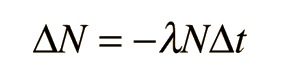where λ is a constant of proportionality (decay constant).

Without any further assumptions, this leads to the exponential radioactive decay result:Show

and also implies that the decay rate and amount of emitted radiation also follow the same type of relationship:Index

 HyperPhysics***** Nuclear R Nave
Go Back

Developing the Decay Expression

Although radioactive decay involves discrete events of nuclear disintegration, the number of events is so large that it can be treated like a continuum and the methods of calculus employed to predict the behavior. The result from the decay probability can be put in the differential form: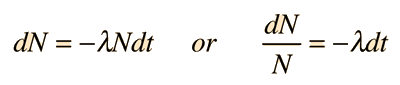This can be integrated directly to give ln N = -λt + C where C is a constant of integration.

Taking the exponent of both sides gives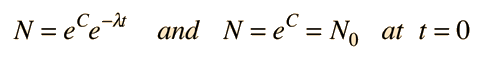so the standard form of the decay equation is:which also applies to all other quantities which are proportional to N.

 Decay by multiple paths
Index

 HyperPhysics***** Nuclear R Nave
Go Back

The rate of radioactive decay is typically expressed in terms of either the radioactive half-life, or the radioactive decay constant. They are related as follows:The decay constant is also sometimes called the disintegration constant. The half-life and the decay constant give the same information, so either may be used to characterize decay. Another useful concept in radioactive decay is the average lifetime. The average lifetime is the reciprocal of the decay constant as defined here.

For example, free neutrons decay with a halflife of about 10.3 minutes. This corresponds to a decay constant of .067/min and an average lifetime of 14.8 minutes or 890 seconds.

For geologic dating processes involving long halflives, like potassium-argon dating, the decay constant is typically expressed in yr-1 and the half-life in years.

Index

 HyperPhysics***** Nuclear R Nave
Go Back

Often a radioactive nucleus will decay by two or more pathways, yielding different final products. If there are two modes, leading to products a and b, then we can represent the decay rates by these two modes by partial decay constants λa and λb defined by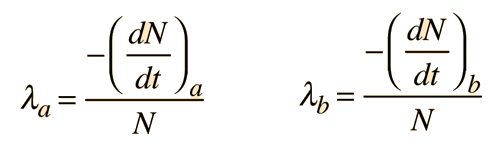Where N is the number of the parent radioactive nuclei. The total decay rate dN/dt is then given by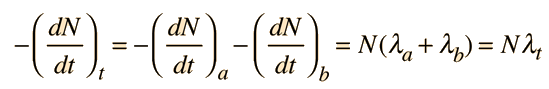The decay is governed by the total decay rate since both are proceeding simultaneously.The number of parent nuclei remaining at time t is then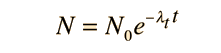and the amounts of the decay products a and b areNote that the individual decay constants λa and λb never appear in the exponential. Both processes continue to proceed and the rate of decay is determined by the sum of their decay constants. This process can be extended to more pathways if all proceed from the same parent nucleus.

If you sought to use the measured population of the isotopes to date a sample, you would not have access to the value N0 of the parent isotope, and would need to recast the above expressions in terms of the current measured value N of the parent. Using product a, the expression for the population Na then becomesand this can be solved algebraically for the time t:This is the approach taken in potassium-argon dating where there are decay modes to both argon and calcium.

Index

Krane, Ch 6

 HyperPhysics***** Nuclear R Nave
Go Back